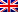#[ECSC Quals 2019] [Crypto 398 – m04r_s1gz]

## Description

Testez la robustesse de notre SecureSigner !

nc challenges.ecsc-teamfrance.fr 2001

## Resolution

Connecting to the server we got:

```Can you beat us and forge a signature in less than 60 seconds?
- modulus n: 23772513176525258116701834760533284490937924906078683821138200234227222212184639522626570329221750795825214530440329226265372134747012808042540058729141411714883175983649546293724661731864019070887307712196599257332952253041916081080359964927430953091436173033599607939453392876843619394783311427121929149821048196093830451856664921553610409424925459851005922219740492445505471981331813952727465089520713865647671269066929136732189463057205377027240601587874433103168160467383065859007442110870502909088872773337503581352030280817983604113951736474669279208356380966385313645970125770633462921080515333558820606489023
- public exponent e: 65537
Please enter a number to sign (or anything else to stop):
```

Each time we connected to the server a new RSA key was generated.
The server returns the modulus and exponent, and the only thing we could do for one minute was to sign numbers or try to sign the provided challenge:

```Please enter a number to sign (or anything else to stop): 1337
Signature: 21788765474516882817850325547933469427810645389313863258683864628880441544560123182182543843962589019547606383318778941236271941702735395506463646098302680207039259185657570657806899441356295033123534898568315445070942584694103819225400802024098435642495244429950254489276303169504526195316673007011101089171610099487254612457571981131594704875293934297856998128052099395994901546322951299979834356178118302875469763735850923308399895322778461497594208137718228886335354575523739104918185533709705406726845221772572944646565251292000769007211231108801657254237394626624929147740995739098617069254626938705206399305341
Please enter a number to sign (or anything else to stop): stop
Enter the signature of the challenge:
```

In order to provide a good signature, we needed to generate n signatures by providing n numbers to sign. WTF? Let’s explain this!
Signature: s = m^d (mod n), with s (signature), m (message to be signed), d (private exponent), n (public modulus)
Verify: s^e = m (mod n), with s (signature), e (public exponent), m (message that was signed), n (public modulus)
As we could compute signature for controlled numbers, we generated n primes numbers to be signed.
primes = primes(range(0, 15000))
For each prime we asked the challenge:
sign_prime1 = challenge(prime1)
When the provided challenge needed to be signed, we splited it in primes factors like:
prime1, prime2, primex, primen = prime_factors(challenge)
Then we calculated the signature as:
signature = (challenge(prime1) * challenge(prime2) * challenge(primex) * challenge(primen)) % N, with N the modulus given on connect

```#!/usr/bin/python3

from pwn import *
from pprint import pprint
from gmpy2 import next_prime
import time

def compute_primes(n):
global primes
i = 0
while i < n:
i = int(next_prime(i))
primes.append(i)

# https://stackoverflow.com/questions/16996217/prime-factorization-list/32608286#32608286
def prime_factors(x):
factorlist=[]
loop=2
while loop<=x:
if x%loop==0:
x//=loop
factorlist.append(loop)
else:
loop+=1
return factorlist

def main():
begin = time.time()
r = remote('challenges.ecsc-teamfrance.fr', 2001)
r.recvuntil('modulus n: ', drop=True)
n = int(r.recvline(keepends=False).decode())
r.recvuntil('exponent e: ', drop=True)
e = int(r.recvuntil('\n', drop=True).decode())
print('N:',n)
print('e:',e)
i = 0
signs = {}
print('Retrieving challenges for 50s...')
while True:
r.recvuntil('to stop): ', drop=True).decode()
i+=1
r.recvuntil('Signature: ', drop=True)
sign = r.recvline(keepends=False).decode()
exectime = time.time()-begin
if exectime > 55:
print('Exec time:',str("{0:.2f}".format(exectime))+'s')
break
r.sendline('gimmetehchallenge!')
r.recvuntil('Here is your challenge: ', drop=True)
chall = r.recvline(keepends=False).decode()
print('Challenge:',chall)
challprimes = prime_factors(int(chall))
signature = 1
for num in challprimes:
try:
signature = signature * int(signs.get(int(num)))
except:
print('No solution found! Trying again...')
r.close()
main()
signature = str(signature % n)
print('sign:',signature)
r.sendline(signature)
r.interactive()

if __name__ == '__main__':
primes = []
compute_primes(15000)
main()

```

Flag was ECSC{f861bb26b32459970ed27dcc804e52c136f48f0c}

## One thought on “[ECSC Quals 2019] [Crypto 398 – m04r_s1gz]”

1.liao says:

gool